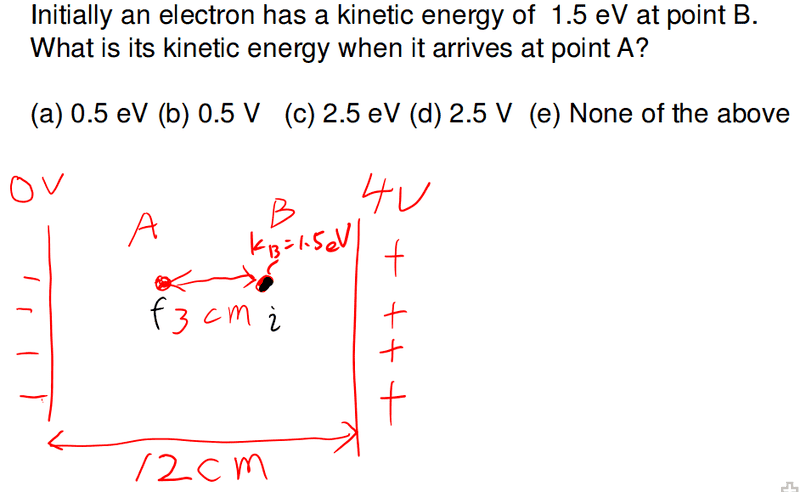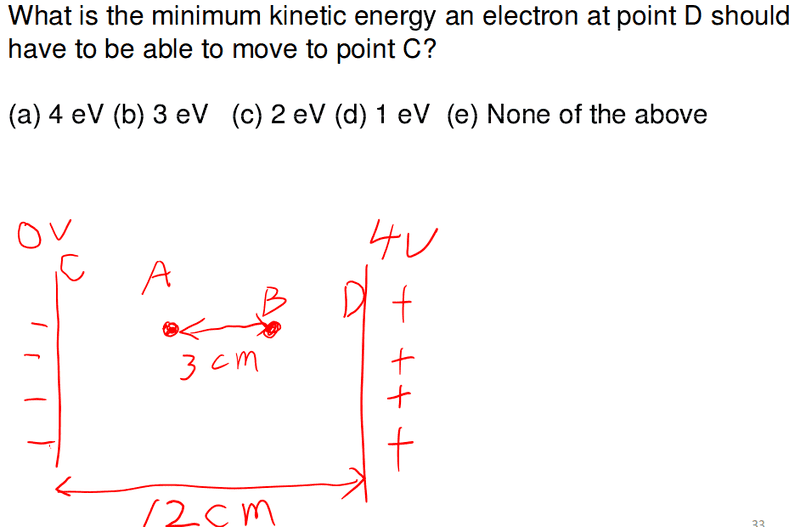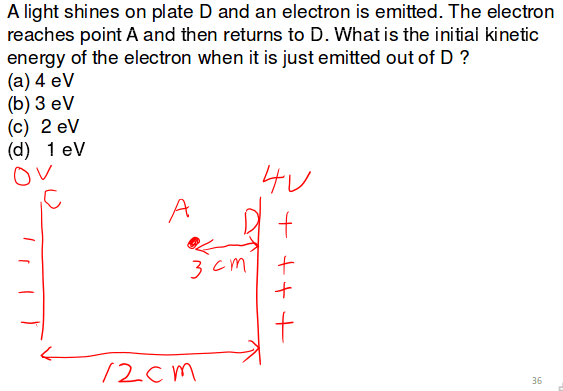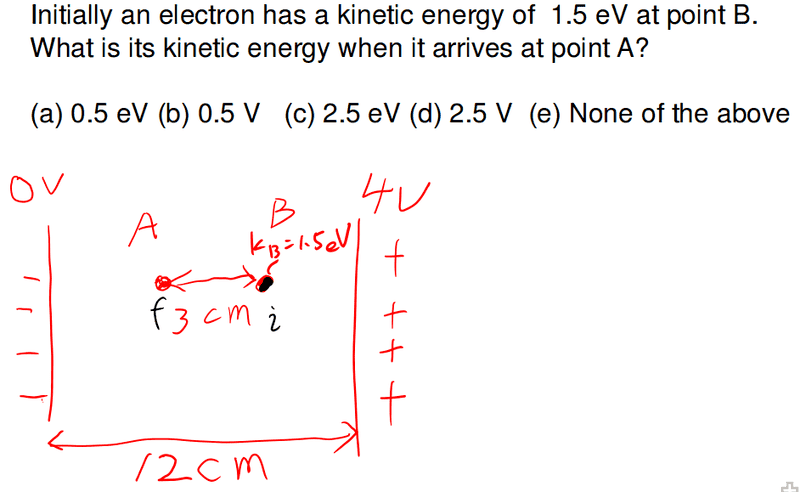# Determing the kinetic energy of an electron

• Turion

## Homework Statement## The Attempt at a Solution

KEi + PEi = KEf
2.4 x 10-19 + e(1) = KEf
KEf = 4.002 x 10-19 J

So option c would be correct, right? Thank you in advance.This one is a trick question, right? It would need 0 kinetic energy because the electric field itself would move the electron to the left until it reaches point C.

This one doesn't even make sense to me. How can the electron go back to plate D if plate D has the higher voltage?Turion said:

## Homework Statement## The Attempt at a Solution

KEi + PEi = KEf
2.4 x 10-19 + e(1) = KEf
KEf = 4.002 x 10-19 J

So option c would be correct, right? Thank you in advance.

No. The electron is repelled by the negative plate so its KE decreases when it moves from B to A.
And it's easier if you work in eV directly.
The potential difference between B and A is 1 V so the change in potential energy is 1eV.

And I think you are supposed to post one problem per topic.